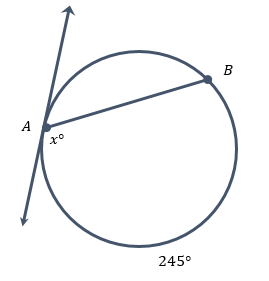HSC Mathematics: Exam Prep & Syllabus Final Exam

Free Practice Test Instructions:

Choose your answer to the question and click 'Continue' to see how you did. Then click 'Next Question' to answer the next question. When you have completed the free practice test, click 'View Results' to see your results. Good luck!

Question 2 2. Simplify the expression shown.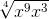Question 3 3. Which graph(s) are indefinite quadratics?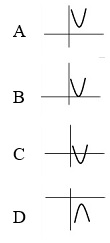Question 4 4. Find f'(x).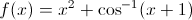Question 7 7. Use Newton's method to find the root of f(x), starting at x=1.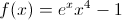Question 8 8. What is the simplified value of the expression below?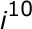Question 10 10. Solve for x using the image as a reference. The perimeter of the triangle is 36 units.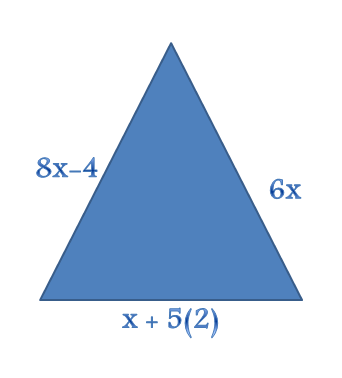Question 11 11. Find the measure of the angle x.